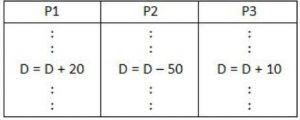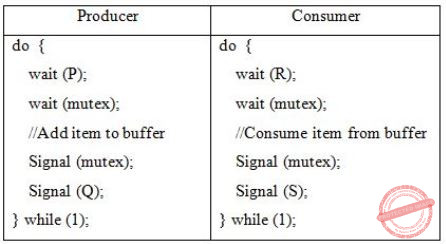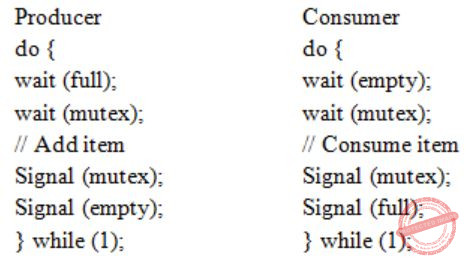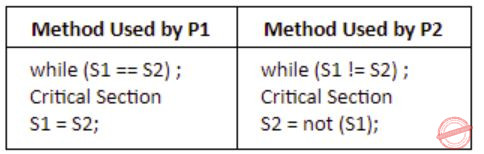## Process-Synchronization

 Question 1

Consider three concurrent processes P1, P2 and P3 as shown below, which access a shared variable D that has been initialized to 100.The process are executed on a uniprocessor system running a time-shared operating system. If the minimum and maximum possible values of D after the three processes have completed execution are X and Y respectively, then the value of Y–X is _______.

 A 10 B 40 C 60 D 80
Operating-Systems       Process-Synchronization       GATE 2019       Video-Explanation
Question 1 Explanation:P1 executes D=D+20, D=120.
P3 executes D=D+10, D=130.
Now, P2 has D=100, executes
D = D-50 = 100-50 = 50
P2 writes D=50 final value. This is minimum.
Next,
P2 reads D=100, executes D = D-50, before that assume P1 & P3 has read D=100.
P2 makes D=50 & writes it.
P1 executes (D=100), D=D+20 & P3 executes D=D+10 gives maximum value D=130.
So, Y - X = 130 - 50 =80.
 Question 2

Consider the following solution to the producer-consumer synchronization problem. The shared buffer size is N. Three semaphores empty, full and mutex are defined with respective initial values of 0, N and 1. Semaphore empty denotes the number of available slots in the buffer, for the consumer to read from. Semaphore full denotes the number of available slots in the buffer, for the producer to write to. The placeholder variables, denoted by P, Q, R and S, in the code below can be assigned either empty or full. The valid semaphore operations are: wait() and signal().Which one of the following assignments to P, Q, R and S will yield the correct solution?

 A P: full, Q: full, R: empty, S: empty B P: empty, Q: empty, R: full, S: full C P: full, Q: empty, R: empty, S: full D P: empty, Q: full, R: full, S: empty
Operating-Systems       Process-Synchronization       GATE 2018       Video-Explanation
Question 2 Explanation:
P=full, Q=empty, R=empty, S=full
Initial: mutex = 1
empty = 0
full = NQuestion 3

A multithreaded program P executes with x number of threads and used y number of locks for ensuring mutual exclusion while operating on shared memory locations. All locks in the program are non-reentrant, i.e., if a thread holds a lock l, then it cannot re-acquire lock l without releasing it. If a thread is unable to acquire a lock, it blocks until the lock becomes available. The minimum value of x and the minimum value of y together for which execution of P can result in a deadlock are:

 A x = 1, y = 2 B x = 2, y = 1 C x = 2, y = 2 D x = 1, y = 1
Operating-Systems       Process-Synchronization       GATE 2017 [Set-1]       Video-Explanation
Question 3 Explanation:
First you have to know multithreading, mutual exclusion and reentrant mutex. The reentrant mutex (recursive mutex, recursive lock) is a particular type of mutual exclusion (mutex) device that may be locked multiple times by the same process/thread, without causing a deadlock.
Here a non-reentrant process can’t own the same lock multiple times, so if the process tries to acquire an already owned lock, it will get blocked, and deadlock will happen.
From the above options x=1 (a single thread) and y=1 (a single lock) deadlock is possible when we consider the given situations in question.
 Question 4

Consider the following proposed solution for the critical section problem. There are n processes: P0...P(n-1). In the code, function pmax returns an integer not smaller than any of its arguments. For all i, t[i] is initialized to zero.

```Code for Pi:

do {

c[i]=1; t[i] = pmax(t,...,t[n-1])+1; c[i]=0;
for every j ≠ i in {0,...,n-1} {
while (c[j]);
while (t[j] != 0 && t[j]<=t[i]);
}
Critical Section;
t[i]=0;
Remainder Section;
} while (true);
```

Which one of the following is TRUE about the above solution?

 A At most one process can be in the critical section at any time B The bounded wait condition is satisﬁed C The progress condition is satisﬁed D It cannot cause a deadlock
Operating-Systems       Process-Synchronization       GATE 2016 [Set-1]       Video-Explanation
Question 4 Explanation:
We will check the four options one by one.
Based on the above code option B, C and D are not satisfied.
We can see that while (t[j] != 0 && t[j] <= t[i]);
because of this condition deadlock is possible when t[j] = = t[i].
Because Progress == no deadlock as no one process is able to make progress by stopping other process.
Bounded waiting is also not satisfied.
In this case both deadlock and bounded waiting to be arising from the same reason as if t[j] = = t[i] is possible then starvation is possible means infinite waiting.
Mutual exclusion is satisfied.
All other processes j started before i must have a value of t[j]) < t[i] as function pMax() return an integer not smaller than any of its arguments.
So if anyone out of the processes j have positive value will be executing in its critical section as long as the condition t[j] > 0 && t[j] <= t[i] within while will persist.
And when this j process comes out of its critical section, it sets t[j] = 0; and next process will be selected in for loop.
So, when i process reaches to its critical section none of the processes j which started earlier before process i is in its critical section.
This ensures that only one process is executing its critical section at a time.
 Question 5

Consider the following two-process synchronization solution.

```Process 0                                     Process 1
---------                                     ---------
Entry: loop while (turn == 1);                Entry: loop while (turn == 0);
(critical section)                            (critical section)
Exit: turn = 1;                               Exit: turn = 0;
```

The shared variable turn is initialized to zero. Which one of the following is TRUE?

 A This is a correct two-process synchronization solution. B This solution violates mutual exclusion requirement. C This solution violates progress requirement. D This solution violates bounded wait requirement.
Operating-Systems       Process-Synchronization       GATE 2016 [Set-2]       Video-Explanation
Question 5 Explanation:
B) Mutual exclusion is satisfied because the value of the turn variable cannot be 0 and 1 at the same time.
So False.
C) Progress means if one process does not want to enter the critical section then it should not stop other process to enter the critical section.
But we can see that if process 0 will not enter the critical section then value of turn will not become 1 and process 1 will not be able to enter critical section.
So progress not satisfied. True.
D) Bounded waiting solution as there is a strict alternation.
So, False.
 Question 6

Consider a non-negative counting semaphore S. The operation P(S) decrements S, and V(S) increments S. During an execution, 20 P(S) operations and 12 V(S) operations are issued in some order. The largest initial value of S for which at least one P(S) operation will remain blocked is _________.

 A 7 B 8 C 9 D 10
Operating-Systems       Process-Synchronization       GATE 2016 [Set-2]       Video-Explanation
Question 6 Explanation:
We can assume the largest initial value of S for which at least one P(S) operation → X
P(S) operation remain in blocked state therefore it will -1.
The negative value of the counting semaphore indicates the number of processes in suspended list (or blocked).
Take any sequence of 20P and 12V operations, at least one process will always remain blocked.
So, X - 20 + 12 = -1
Here P(S) = 20 and V(S) = 12
X = 7
 Question 7

The following two functions P1 and P2 that share a variable B with an initial value of 2 execute concurrently.

```P1()
{
C = B – 1;
B = 2*C;
}

P2()
{
D = 2 * B;
B = D - 1;
}```

The number of distinct values that B can possibly take after the execution is

 A 3 B 4 C 5 D 6
Operating-Systems       Process-Synchronization       GATE 2015 [Set-1]
Question 7 Explanation:
If we execute P2 process after P1 process, then B = 3
If we execute P1 process after P2 process, then B = 4
If we did preemption between P1 & P2 processes, then B = 2 (Preemption have done from P1 to P2) or B = 3 (Preemption have done from P2 to P1). So, among 2 & 3 values, only one value will be saved in B. So, total no. of distinct values that B can possibly take after the execution is 3.
 Question 8

Consider the procedure below for the Producer-Consumer problem which uses semaphores:

```semaphore n=0;
semaphore s=1;
void producer()          void consumer()
{                        {
while(true)              while(true)
{                        {
produce();               semWait(s);
semWait(s);              semWait(n);
semSignal(s);            semSignal();
semSignal(n);            consume();
}                        }
}                         }
```

Which one of the following is TRUE?

 A The producer will be able to add an item to the buffer, but the consumer can never consume it. B The consumer will remove no more than one item from the buffer. C Deadlock occurs if the consumer succeeds in acquiring semaphore s when the buffer is empty. D The starting value for the semaphore n must be 1 and not 0 for deadlock-free operation.
Operating-Systems       Process-Synchronization       GATE 2014 [Set-2]
Question 8 Explanation:
Answer is (C), because when consumer first access the semaphore 's' it will down (s) and make 's'=0, but for semaphore(n), it has to wait for producer to make it 1 but as for producer it can't access the critical section because the value of s=0, so semWait(s) will not work. So there will be deadlock.
 Question 9

Fetch_And_Add(X,i) is an atomic Read-Modify-Write instruction that reads the value of memory location X, increments it by the value i, and returns the old value of X. It is used in the pseudocode shown below to implement a busy-wait lock. L is an unsigned integer shared variable initialized to 0. The value of 0 corresponds to lock being available, while any non-zero value corresponds to the lock being not available.

```  AcquireLock(L){
L = 1;
}
ReleaseLock(L){
L = 0;
}```

This implementation

 A fails as L can overflow B fails as L can take on a non-zero value when the lock is actually available C works correctly but may starve some processes D works correctly without starvation
Operating-Systems       Process-Synchronization       GATE 2012
Question 9 Explanation:
Check the loop first:
L = 1; // A waiting process can be here just after
// the lock is released, and can make L = 1.
Assume P1 executes until while condition and preempts before executing L =1. Now P2 executes all statements, hence L = 0. Then P1 without checking L it makes L = 1 by executing the statement where it was preempted.
It takes a non-zero value (L=1) when the lock is actually available (L = 0). So option B.
 Question 10

Consider the methods used by processes P1 and P2 for accessing their critical sections whenever needed, as given below. The initial values of shared boolean variables S1 and S2 are randomly assigned.Which one of the following statements describes the properties achieved?

 A Mutual exclusion but not progress B Progress but not mutual exclusion C Neither mutual exclusion nor progress D Both mutual exclusion and progress
Operating-Systems       Process-Synchronization       GATE 2010
Question 10 Explanation:
In this mutual exclusion is satisfied because at any point of time either S1 = S2 or S1 ≠ S2, but not both. But here progress is not satisfied because suppose S1 = 1 and S2 = 0 and P1 is not interested to enter into critical section but P2 wants to enter into critical section, and P2 will not be able to enter, because until P1 will not enter critical section, S1 will not become equal to S2. So if one process is not interested in entering the critical section, it will not allow the other process to enter the critical section which is interested. So progress is not satisfied.
 Question 11

The following program consists of 3 concurrent processes and 3 binary semaphores.The semaphores are initialized as S0 = 1, S1 = 0, S2 = 0.How many times will process P0 print '0'?

 A At least twice B Exactly twice C Exactly thrice D Exactly once
Operating-Systems       Process-Synchronization       GATE 2010
Question 11 Explanation:
S0=1
S1=0
S2=0
P0 enters the critical section first,
prints (‘0’)
releases S1,S2(i.e., S1=1 & S2=1)
Now P1 & P2 both can enter critical section releases S0 & prints (‘0’)
This process continues, hence the number of zero’s printed ≥2.
 Question 12

The enter_CS() and leave_CS() functions to implement critical section of a process are realized using test-and-set instruction as follows:

```void enter_CS(X)
{
while test-and-set(X) ;
}
void leave_CS(X)
{
X = 0;
}```

In the above solution, X is a memory location associated with the CS and is initialized to 0. Now consider the following statements:

I. The above solution to CS problem is deadlock-free.
II. The solution is starvation free.
III. The processes enter CS in FIFO order.
IV More than one process can enter CS at the same time.

Which of the above statements is TRUE?

 A I only B I and II C II and III D IV only
Operating-Systems       Process-Synchronization       GATE 2009
Question 12 Explanation:
The given solution is the basic test-and-set solution. So there is no possibility of getting deadlock.
It is not using any queue to avoid starvation and there is no use of FIFO.
In CS, only one process can enter.
 Question 13

The P and V operations on counting semaphores, where s is a counting semaphore, are defined as follows:

```P(s): s =  s - 1;
if (s < 0) then wait;
V(s): s = s + 1;
if (s <= 0) then wakeup a process waiting on s;```

Assume that Pb and Vb the wait and signal operations on binary semaphores are provided. Two binary semaphores Xb and Yb are used to implement the semaphore operations P(s) and V(s) as follows:

```P(s): Pb(Xb);
s = s - 1;
if (s < 0) {
Vb(Xb) ;
Pb(Yb) ;
}
else Vb(Xb);

V(s): Pb(Xb) ;
s = s + 1;
if (s <= 0) Vb(Yb) ;
Vb(Xb) ;```

The initial values of Xb and Yb are respectively

 A 0 and 0 B 0 and 1 C 1 and 0 D 1 and 1
Operating-Systems       Process-Synchronization       GATE 2008
Question 13 Explanation:
xb must be 1 because both P(s) operation and V(s) operation perform Pb(xb) first. So if xb=0, then all the process performing these operations will be blocked.
Yb must be '0' initially, because if Yb is '1' initially then two process can be in critical section at the same time.
 Question 14

Two processes, P1 and P2, need to access a critical section of code. Consider the following synchronization construct used by the processes:

```  /* P1 */
while (true) {
wants1 = true;
while (wants2 == true);
/* Critical
Section */
wants1=false;
}
/* Remainder section */

/* P2 */
while (true) {
wants2 = true;
while (wants1==true);
/* Critical
Section */
wants2 = false;
}
/* Remainder section */ ```

Here, wants1 and wants2 are shared variables, which are initialized to false. Which one of the following statements is TRUE about the above construct?

 A It does not ensure mutual exclusion. B It does not ensure bounded waiting. C It requires that processes enter the critical section in strict alternation. D It does not prevent deadlocks, but ensures mutual exclusion.
Operating-Systems       Process-Synchronization       GATE 2007
Question 14 Explanation:
First of all it can be easily seen that mutual exclusion is satisfied.
Now, if in P1
wants1 = true ;
executed and preempted and now if in P2
wants2 = true ;
executed.
Then the deadlock situation will be created because both will fall into infinite loop.
 Question 15

The atomic fetch-and-set x, y instruction unconditionally sets the memory location x to 1 and fetches the old value of x n y without allowing any intervening access to the memory location x. consider the following implementation of P and V functions on a binary semaphore S.

```void P (binary_semaphore *s)
{
unsigned y;
unsigned *x = &(s->value);
do
{
fetch-and-set x, y;
}
while (y);
}
void V (binary_semaphore *s)
{
S->value = 0;
}```

Which one of the following is true?

 A The implementation may not work if context switching is disabled in P B Instead of using fetch-and-set, a pair of normal load/store can be used C The implementation of V is wrong D The implementation of V is wrong
Operating-Systems       Process-Synchronization       GATE 2006
Question 15 Explanation:
A) This is correct because implementation might not work if context switching is disabled in P, then process which is currently blocked may never give control to the process which might eventually execute v. So context switching is must.
B) If we use normal load and store instead of Fetch and Set, then there can be chance that more than one process sees S.value as 0 and then mutual exclusion will not be satisfied. So wrong.
C) Here we are setting S→value to 0, which is correct. This option that's why wrong.
D) Only one process can be in critical section at any time. So this option is wrong.
 Question 16

Barrier is a synchronization construct where a set of processes synchronizes globally i.e. each process in the set arrives at the barrier and waits for all others to arrive and then all processes leave the barrier. Let the number of processes in the set be three and S be a binary semaphore with the usual P and V functions. Consider the following C implementation of a barrier with line numbers shown on left.

```void barrier (void) {
1:   P(S);
2:   process_arrived++;
3.   V(S);
4:   while (process_arrived !=3);
5:   P(S);
6:   process_left++;
7:   if (process_left==3) {
8:      process_arrived = 0;
9:      process_left = 0;
10:  }
11:  V(S);
}```

The variables process_arrived and process_left are shared among all processes and are initialized to zero. In a concurrent program all the three processes call the barrier function when they need to synchronize globally.

The above implementation of barrier is incorrect. Which one of the following is true?

 A The barrier implementation is wrong due to the use of binary semaphore S B The barrier implementation may lead to a deadlock if two barriers in invocations are used in immediate succession C Lines 6 to 10 need not be inside a critical section D The barrier implementation is correct if there are only two processes instead of three
Operating-Systems       Process-Synchronization       GATE 2006
Question 16 Explanation:
If process-arrived is because greater than 3. Then there is no possibility to be 3.
 Question 17

Barrier is a synchronization construct where a set of processes synchronizes globally i.e. each process in the set arrives at the barrier and waits for all others to arrive and then all processes leave the barrier. Let the number of processes in the set be three and S be a binary semaphore with the usual P and V functions. Consider the following C implementation of a barrier with line numbers shown on left.

```void barrier (void) {
1:   P(S);
2:   process_arrived++;
3.   V(S);
4:   while (process_arrived !=3);
5:   P(S);
6:   process_left++;
7:   if (process_left==3) {
8:      process_arrived = 0;
9:      process_left = 0;
10:  }
11:  V(S);
} ```

The variables process_arrived and process_left are shared among all processes and are initialized to zero. In a concurrent program all the three processes call the barrier function when they need to synchronize globally.

Which one of the following rectifies the problem in the implementation?

 A Lines 6 to 10 are simply replaced by process_arrived-- B At the beginning of the barrier the first process to enter the barrier waits until process_arrived becomes zero before proceeding to execute P(S). C Context switch is disabled at the beginning of the barrier and re-enabled at the end. D The variable process_left is made private instead of shared.
Operating-Systems       Process-Synchronization       GATE 2006
Question 17 Explanation:
If process-arrived is becomes zero then process_left becomes zero. Then deadlock may resolves.
 Question 18

Consider two processes P1 and P2 accessing the shared variables X and Y protected by two binary semaphores SX and SY respectively, both initialized to 1. P and V denote the usual semaphone operators, where P decrements the semaphore value, and V increments the semaphore value. The pseudo-code of P1 and P2 is as follows:

```P1 :
While true do {
L1 : ................
L2 : ................
X = X + 1;
Y = Y - 1;
V(SX);
V(SY);
}
P2 :
While true do {
L3 : ................
L4 : ................
Y = Y + 1;
X = Y - 1;
V(SY);
V(SX);
}```

In order to avoid deadlock, the correct operators at L1, L2, L3 and L4 are respectively

 A P(Sy), P(Sx); P(Sx), P(Sy) B P(Sx), P(Sy); P(Sy), P(Sx) C P(Sx), P(Sx); P(Sy), P(Sy) D P(Sx), P(Sy); P(Sx), P(Sy)
Operating-Systems       Process-Synchronization       GATE 2004
Question 18 Explanation:
Option D:
P1 : line 1
P2 : line 3 (block require Sx)
P1 : line 2
P2 : line 4 (still in block state)
P1 : execute CS, the push up the value of Sx.
P2 : line 3 line 4 (block require Sy)
P1 : push up Sy
P2 : line 4 get Sy and enter into CS
P2 : start execution.
 Question 19

Suppose we want to synchronize two concurrent processes P and Q using binary semaphores S and T. The code for the processes P and Q is shown below.

```Process P:
while (1) {
W:
print '0';
print '0';
X:
}

Process Q:
while (1) {
Y:
print '1';
print '1';
Z:
}```

Synchronization statements can be inserted only at points W, X, Y and Z.

Which of the following will always lead to an output staring with '001100110011'?

 A P(S) at W, V(S) at X, P(T) at Y, V(T) at Z, S and T initially 1 B P(S) at W, V(T) at X, P(T) at Y, V(S) at Z, S initially 1, and T initially 0 C P(S) at W, V(T) at X, P(T) at Y, V(S) at Z, S and T initially 1 D P(S) at W, V(S) at X, P(T) at Y, V(T) at Z, S initially 1, and T initially 0
Operating-Systems       Process-Synchronization       GATE 2003
Question 19 Explanation:
Option B is correct.
Process P1 will be executed first and then Process P2 can be executed next.
At the process P: W=P(S)
X=V(T)
At the process Q: Y=P(T)
Z=V(S)
Here, S=1, T=0 then the process P executes first and then Q, and both can run on process alternate way start with P.
There are 19 questions to complete.

Register Now